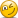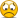Exam FeedbackFRM Part 1 (November 2014) Exam Feedback

New Member
There was a 2-question set about hedging with futures and options. The first question is about the value of option contracts if I remember correctly, and the second one is what you should do if price increases and other factors stay the same. What is your take?
I also have no clue of the answer for the question about projected forward rates and swap rates for 6 months, 1 year and 1.5 years.

ChBurger

New Member
Hi all,

I did the March 2014 part I and the November 2014 part I. Compared to the March I have a better feeling about the exam, although I thought there were much less questions which required calculation compared to March. So my opinion was that this exam was harder compared to the March 2014 exam.

Gini question I did not expect. Also question about Indian gold was not clear (cannot remember the exact question). Indeed question about Wholesale etc. was a random guess for me too. Also the answer to the question about assets 50 USD/50 GBP and liabilities 100 USD did not completely match the answers. I guess my answer was 9%.

dico

New Member
Subscriber
hi ,
It was my first FRM exam, and it was harder than expected. i used Schweser-kaplan to get ready but obviously it was not enough. i will take BT in addition for the part 2, for the best, or /and Part 1 again for the worst.
if i remember questions i will post it, but for the time being some kind of magic spell reset my memory..or i get older...

good luck to everyone,

[email protected]

New Member
Something i remember
1)question around capm ratios, which of the following doesn't consider something correlation(which shud be beta)- treynor,Sharpe,Jensen,information ratio..I answered Sharpe.
2)question related to adjusted coefficient of determination- r2...I was stupid to select it can be negative.
3)one around treasury note...I was blank and randomly selected 985 or something.
4)garp code of conduct question was quite straight forward
5)bull put spread related question- max profit I think
6)Vega for a delta neutral,positive gamma exposure..I was not sure but guessed something which indicates low impact
7)impact of increasing n from 250 to 1000 on CI in a Monte Carlo Sim..I couldnt remember and answered reduce by 1/4
8)American option exercise related....when to exercise?
9)probability of exceeding 99% var 2 times in a 90 day HP. I always go wrong here.pls help me to understand this.
10)gini coefficient ordering
11)question on identifying CTD
12)allied Irish bank and barrings similarity
13)Lease rate calculation given future,spot,risk free rate values
14)negative tail and -/+ve excess kurtosis
15)garch mean reversion related...I chose one with a+b higher to take longer to converge
16)ES and VaR values..I used sub additivity for ES
17)question around multicollinearity meaning
18)CAPM Assumptions
19)ES shortfall VaR calculation..I took average of all numbers to the left of cut off point
20)one related to sample mean and std deviation
21)related to r2 calculation
22)t dist property in relation to ND
23)swap value given jpy and USD positions
24)no of futures contract related
25)percentage increase- DV01/DURATION etc
26)probability of default calculation
27)american put minimum value
28)short the basis question- no and long/short
29)adjusted exposure and expected loss calc or unexpected loss( don't remember)
30)something related to hedging using intermediate rates for position with long and short term rates
31)EWMA related...out of 4 eqns which one gives less/more weight to recent return
32)forward rate calculation

Last edited:

alinafrmmaster

New Member
adjusted R2 can be negative, so that was the right answerso you are not stupid

jonkong

New Member
Subscriber
Something i remember
14)negative tail and -ve excess kurtosis

Was it really negative excess kurtosis? I could've sworn it was positive.. hence I think I answered leptokurtic.

If it was negative afterall then that's another mark stolen by the FRM fairy...

ducanh1409

New Member
adjusted R2 can be negative, so that was the right answerso you are not stupid
I believe that adjusted R2 can be negative. However, as K increase (number of independent variable). Adjust R Square also increase. So Adj R Square increase is the right answer ._ American Call is optimal to excercise if D is large enough
_ I believe its provide Kurtosis number (not excess kurtosis) and it <3. In A,B,C,D choice there ais "excess kurtosis" word though

Last edited:

jonkong

New Member
Subscriber
I had been studying for Part 1 for roughly 5 months going through Schweser notes and past years stretching back to 1997. even found some materials from other third party providers and did those exercises too. After all that, I felt pretty confident cruising through towards exam day.

In the end, I found myself finishing up just as the proctor announced that time was up! I usually get to have some buffer time to go through my previous answers but found no solace this time. All I can do is hope for the best at this point - I figure about 25 or so questions I wasn't too confident in.

jonkong

New Member
Subscriber
I believe that adjusted R2 can be negative. However, as K increase (number of independent variable). Adjust R Square also increase. So Adj R Square increase is the right answer .Correct me if I'm wrong but... if k increases, the fractional term should increase, thus making Adj R squared = 1 - 'a larger value' => Adj R squared decreases; keeping all else constant.

ducanh1409

New Member
Ok I wrong at this question againsad

Goldfish3

New Member
Was it really negative excess kurtosis? I could've sworn it was positive.. hence I think I answered leptokurtic.

If it was negative afterall then that's another mark stolen by the FRM fairy...

I explicitly remember tgus question as being skewness 0.8 and excess kurtosis 1.9. So tail right and more extreme observations

ducanh1409

New Member
I was panic in the last 50 question because I only have 1h40 left. So i was not sure i have committed any more mistakes

New Member
There was a 2-question set about hedging with futures and options (option price is 0.4 if I remember correctly, and hedge ratio is 0.9). The first question is about the value of option contracts if I remember correctly, and the second one is what you should do if price increases and other factors stay the same. What is your take?
I also have no clue of the answer for the question about projected forward rates and swap rates for 6 months, 1 year and 1.5 years.
any help with these two questions?

jonkong

New Member
Subscriber
any help with these two questions?

for the first question I started off with hedge ratio * portfolio value/futures value, then started multiplying/dividing randomly and i think got an answer similar to 350,000. could be right/wrong here. the second part i think i answered nothing happens.

for projected forward rates, i attemped to use the swap rates to calculate a forward rate and concluded that the 1y and 1.5y fwd rates were not correct.

[email protected]

New Member
I explicitly remember tgus question as being skewness 0.8 and excess kurtosis 1.9. So tail right and more extreme observations
May be I m wrong..I don't remember +ve or - I think.

[email protected]

New Member
How many questions u think we shud get rite to pass? I m so stressed out....

jonkong

New Member
Subscriber
With regards to a question about an analyst backtesting a 99% VaR, and to calculate the probablity of 2 exceedences:

I finally figured this out at my desk. The key is to use the binomial equation where probability of exceedence, p = 0.01 and X = exceedence

P(X=2) = 90C2 x (0.01)^2 x (1 - 0.01)^88 = 16.54% (Answer A)

By the roll of an imaginary die, I managed to answer this correctly. It looks like one of those questions where GARP decided to take two AIMs in different sections and combine it into one question to test candidates.

David Harper CFA FRM

David Harper CFA FRM
Staff member
Subscriber
Hi @jonkong did you encounter this backtest question in a Part 1 exam? (This is a P1 thread. "Backtest" does not appear in P1 syllabus to my knowledge, it is a P2 topic)

jonkong

New Member
Subscriber
Hi @jonkong did you encounter this backtest question in a Part 1 exam? (This is a P1 thread. "Backtest" does not appear in P1 syllabus to my knowledge, it is a P2 topic)

Hi David, I did indeed! Pretty sure I came out of the P1 exam on Saturday haha. I was surprised to see such a question myself as it was the first time in all the practice/exercise questions I've burned through

New Member
With regards to a question about an analyst backtesting a 99% VaR, and to calculate the probablity of 2 exceedences:

I finally figured this out at my desk. The key is to use the binomial equation where probability of exceedence, p = 0.01 and X = exceedence

P(X=2) = 90C2 x (0.01)^2 x (1 - 0.01)^88 = 16.54% (Answer A)

By the roll of an imaginary die, I managed to answer this correctly. It looks like one of those questions where GARP decided to take two AIMs in different sections and combine it into one question to test candidates.
This question was a purely probability question for me. I basically ignored the "backtesting" term and just calculated just as you demonstrated.

Replies
338
Views
75K
Replies
57
Views
16K
Replies
44
Views
10K
Replies
158
Views
34K